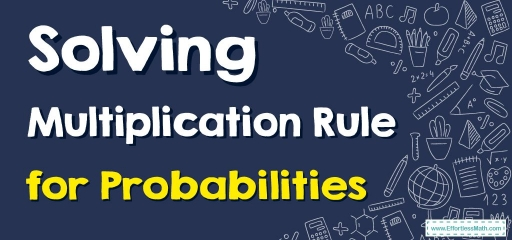# How to Solve Multiplication Rule for Probabilities?

The law of multiplication states that the probability that $$A$$ and $$B$$ occur is equal to the probability that $$A$$ occurs times the conditional probability that $$B$$ occurs, given that we know $$A$$ has already occurred.Using the multiplication rule, you can calculate the probability that events $$A$$ and $$B$$ occur jointly when you know the probability of event $$A$$ and event $$B$$ occurring separately.

## A step-by-step guide to multiplication rule for probabilities

Before we go into the laws of probability, let’s define a few terms:

• If two events cannot happen at the same moment, they are mutually exclusive or discontinuous.
• A conditional probability is a likelihood that event $$A$$ will occur if event $$B$$ has already occurred. The symbol $$P(A|B)$$ represents the conditional probability of event $$A$$ given event $$B$$.
• An event’s complement is the event that does not occur. $$P(A’)$$ indicates the chance that event A will not occur.
• The probability of both events $$A$$ and $$B$$ occurring is the probability of $$A$$ and $$B$$ intersecting. The likelihood of events $$A$$ and $$B$$ intersecting is indicated by $$P(A∩ B)$$. $$P(A∩ B) = 0$$ if events $$A$$ and $$B$$ are mutually exclusive.
• The probability that events $$A$$ or $$B$$ occur is the probability of the union of $$A$$ and $$B$$. The probability of the union of events $$A$$ and $$B$$ is indicated by $$P(A ∪ B)$$.
• Events $$A$$ and $$B$$ are dependent if the occurrence of event $$A$$ alters the likelihood of event $$B$$. Events $$A$$ and $$B$$, on the other hand, are independent if the occurrence of event $$A$$ does not affect the probability of event $$B$$.

### Multiplication rule

In probability theory, the law of multiplication states that given that event $$A$$ has occurred, the probability that events $$A$$ and $$B$$ will both occur is equal to the probability that event $$A$$ will occur multiplied by the probability that event $$B$$ will occur.

$$\color{blue}{P(A∩B)=P(B)⋅P(A|B)}$$

By shifting the roles of $$A$$ and $$B$$, we can also write the law:

$$\color{blue}{P(A∩B)=P(A)⋅P(B|A)}$$

The general multiplication rule is obtained by multiplying both sides of the definition of conditional probability by the denominator. That is, in the equation:

$$\color{blue}{ P(A|B) =\frac{P(A∩B)}{P(B)}}$$

The rule is useful when we know both $$P(A)$$ and $$P(B|A)$$ or both $$P(B)$$ and $$P(A|B)$$.

### Multiplication Rule for Probabilities – Example 1:

A jar contains $$5$$ red marbles and $$4$$ black marbles. Two marbles are pulled from the jar without replacement. What is the probability that both marbles are black?

If $$A =$$ the event that the first marble is black; and if $$B =$$ the event that the second marble is black. We know the following:

Initially, there are $$9$$ marbles in the jar, $$4$$ of which are black. Therefore, $$P(A)=\frac{4}{9}$$.

After the first selection, there are $$8$$ marbles in the jar, $$3$$ of which are black. Therefore, $$P(B|A)=\frac{3}{8}$$.

Then, use this formula: $$\color{blue}{P(A∩B)=P(A)⋅P(B|A)}$$

$$P(A∩B) = \frac{4}{9} \times \frac{3}{8} =\frac{4 \times 3 }{9 \times 8}=\frac{12}{72}=\frac{1}{6}$$

## Exercises for Multiplication Rule for Probabilities

A social swimming team has $$150$$ members. Seventy-five members are advanced swimmers. Forty-seven members are intermediate swimmers. The rest of the swimmers are novices. Forty advanced swimmers practice three times a week. Thirty of the intermediate swimmers practice three times a week. Ten novice swimmers practice three times a week. Suppose a member of a swimming team is randomly selected.

1. What is the probability that a member is a novice swimmer?
2. What is the probability that a member exercises four times a week?
3. What is the probability that the member is an advanced swimmer and practices three times a week?
4. What is the probability that a member is an advanced swimmer and an intermediate swimmer?
1. $$\color{blue}{\frac{28}{150}}$$
2. $$\color{blue}{\frac{80}{150}}$$
3. $$\color{blue}{\frac{40}{150}}$$
4. $$\color{blue}{P=0}$$

### What people say about "How to Solve Multiplication Rule for Probabilities? - Effortless Math: We Help Students Learn to LOVE Mathematics"?

No one replied yet.

X
30% OFF

Limited time only!

Save Over 30%

SAVE $5 It was$16.99 now it is \$11.99